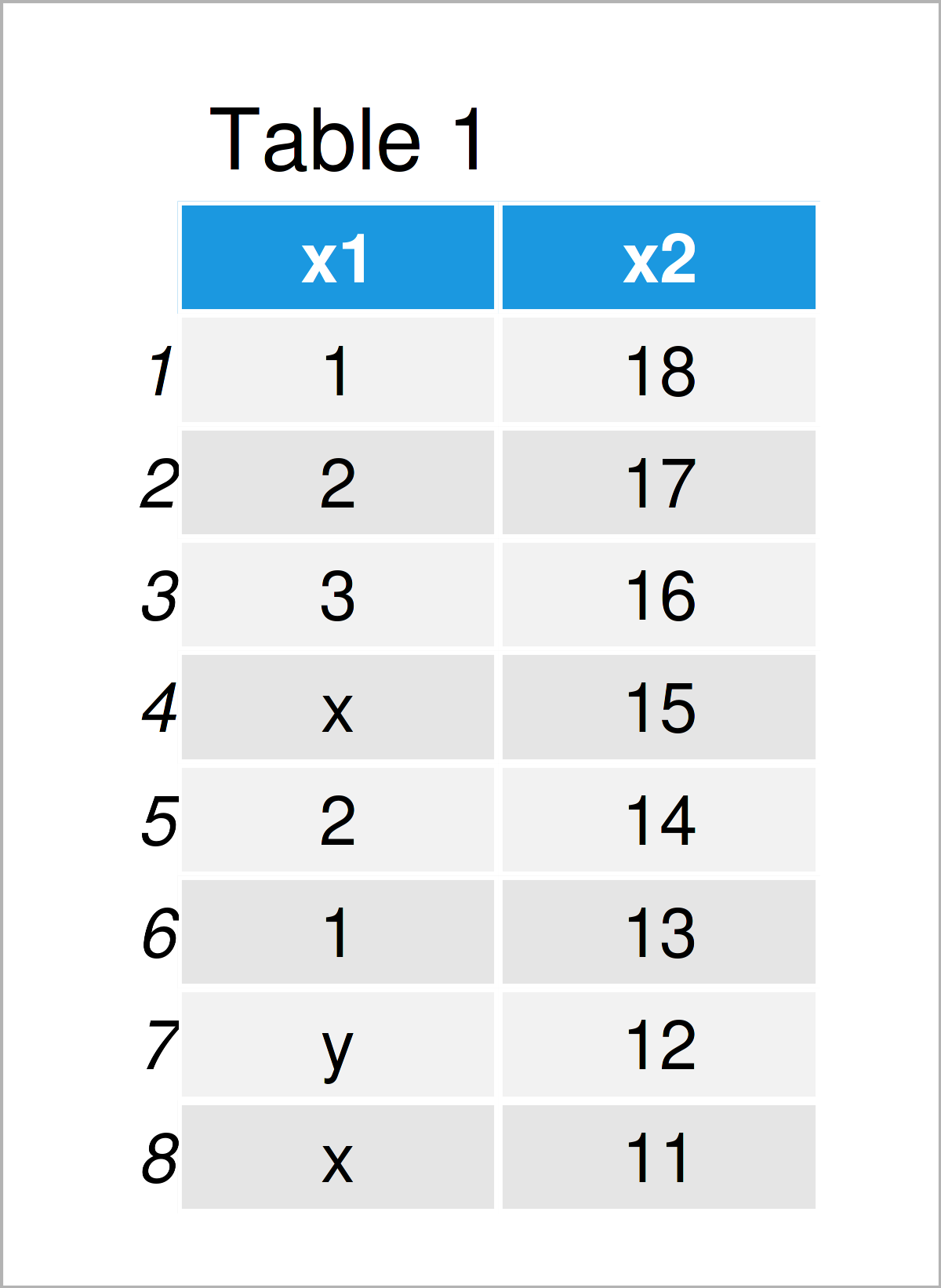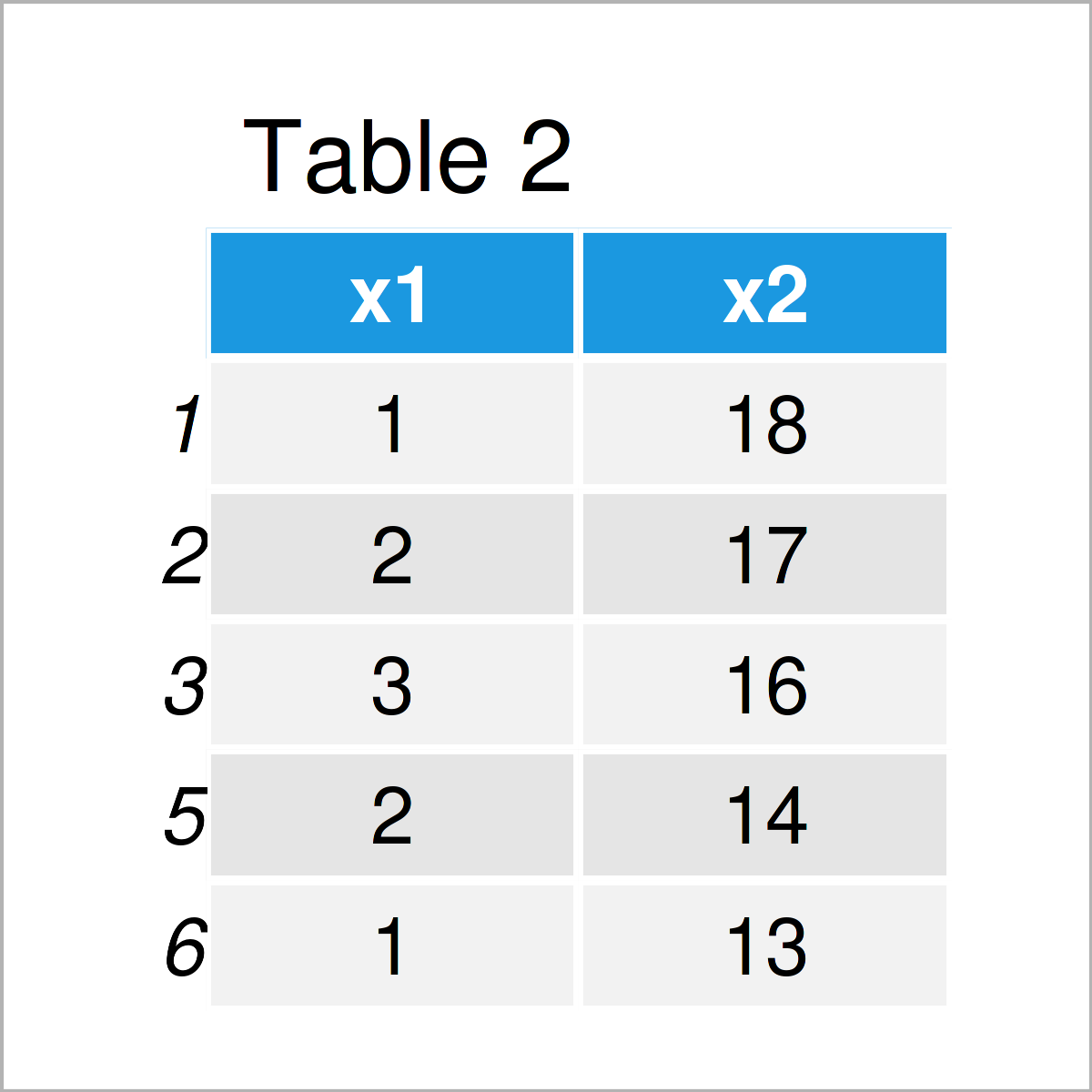# Remove Rows with Non-Numeric Characters in R (Example)

This tutorial demonstrates how to drop data frame rows with non-numeric characters in a certain column in the R programming language.

Let’s just jump right in!

## Creation of Example Data

First, let’s create some example data:

```data <- data.frame(x1 = c(1:3, "x", 2:1, "y", "x"),  # Create example data frame
x2 = 18:11)
data                                                 # Print example data frame```Table 1 illustrates the output of the RStudio console that has been returned after executing the previous R code and shows that the example data is made up of eight rows and two columns.

## Example: Keep Only Rows with Numbers in Certain Column Using as.numeric() & is.na() Functions

This example illustrates how to delete all rows where a specific column contains non-numeric values.

For this task, we can apply the as.numeric and is.na functions as shown below:

```data_new <- data[!is.na(as.numeric(data\$x1)), ]      # Delete rows
data_new                                             # Print data frame subset```As shown in Table 2, we have created a new data frame subset by executing the previous R programming code. This subset contains only those rows where the column x1 contained numeric values.

Note that the previous R code also returns the warning message “In `[.data.frame`(data, !is.na(as.numeric(data\$x1)), ) : NAs introduced by coercion“. That is because the as.numeric function converts non-numeric values to NA.

## Video, Further Resources & Summary

I have recently published a video on my YouTube channel, which illustrates the contents of this article. You can find the video below.

Furthermore, you might want to read the related articles on my website.

You have learned in this article how to remove data frame rows with non-numeric characters in a particular column in R programming. Please let me know in the comments below, in case you have further comments or questions.

Subscribe to the Statistics Globe Newsletter